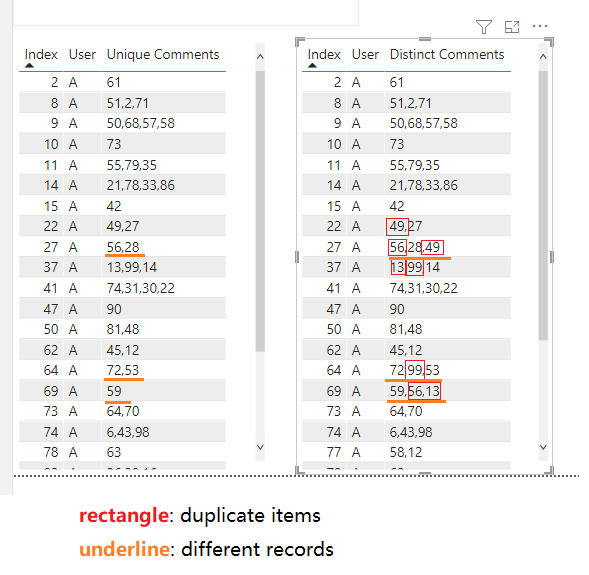cancel
Showing results for
Did you mean:## Comparison of the text string with multiple elements

We often analyse with text values, the DAX text functions will help us analyse these values.

Sometime we may need to do some advanced text comparison or accurately analyse the internal elements (e.g. compare with current and previous record contexts, get the popular items from field values) and the basic DAX text function may not be suitable for these situations.

If you have similar requirement, please check the following scenario.

Usages:

Sample table with a list of records of users and comments.#1, List the distinct elements of each user.

We can simple use CONCATENATEX function to merge these comment values. However, the merged results will include the duplicate values.

``Formula = CONCATENATEX ( VALUES ( T1[Comment] ), [Comment], "," )``For this scenario, you need to split each element in each comment to a list, and then we can remove duplicate value from these records.

Trick: use GENERATESERIES and PATH functions to extract the element to list.

``````Distinct Comments =
VAR merged =
CONCATENATEX ( VALUES ( T1[Comment] ), [Comment], "," )
VAR _path =
SUBSTITUTE ( merged, ",", "|" )
VAR list =
SELECTCOLUMNS (
GENERATESERIES ( 1, PATHLENGTH ( _path ), 1 ),
"Item", PATHITEM ( _path, [Value] )
)
RETURN
CONCATENATEX (
DISTINCT ( list ),
[Item],
","
)``````Comment:

1. Path function can recognize each item in text string that stored with delimiter '|'. (I used the SUBSTITUTE to replace original comma '|' to help Path function to recognize these elements)
2. GENERATESERIES function can generate a table with specific start end range and interval.
3. For the generated table, the End range should be the element count (pathlength) and each item can be matched and extracted by PATHITEM function.

#2, Show the most popular comment for each user. Use above methods to get the list of comment, then you can use groupby function to summary these records and show the count.

Trick: SUMMARIZE and GROUPBY functions can be used to aggregate table records.

``````Most popular comment =
VAR merged =
CONCATENATEX ( VALUES ( T1[Comment] ), [Comment], "," )
VAR _path =
SUBSTITUTE ( merged, ",", "|" )
VAR list =
SELECTCOLUMNS (
GENERATESERIES ( 1, PATHLENGTH ( _path ), 1 ),
"Item", PATHITEM ( _path, [Value] )
)
var summary=GROUPBY(list,[Item],"Count",COUNTX(CURRENTGROUP(),[Item]))
RETURN
CONCATENATEX (
FILTER(summary,[Count]>1),
[Item],
","
)``````#3, Remove duplicate comment that was displayed in history records.

Reproduce the above steps to get the current list, use the current index as condition to lookup and extract the previous list.

Trick: EXCEPT function be used to compare two table/list and remove the right item from the left one.

``````Unique Comments =
VAR currIndex =
MAX ( T1[Index] )
CONCATENATEX ( VALUES ( T1[Comment] ), [Comment], "," )
VAR _currPath =
SUBSTITUTE ( currComments, ",", "|" )
VAR currlist =
SELECTCOLUMNS (
GENERATESERIES ( 1, PATHLENGTH ( _currPath ), 1 ),
"Item", PATHITEM ( _currPath, [Value] )
)
CONCATENATEX (
CALCULATETABLE (
VALUES ( T1[Comment] ),
FILTER ( ALLSELECTED ( T1 ), [Index] < currIndex ),
VALUES ( T1[User] )
),
[Comment],
","
)
VAR _prevPath =
SUBSTITUTE ( prevComments, ",", "|" )
RETURN
IF (
_prevPath <> BLANK (),
VAR prevlist =
SELECTCOLUMNS (
GENERATESERIES ( 1, PATHLENGTH ( _prevPath ), 1 ),
"Item", PATHITEM ( _prevPath, [Value] )
)
RETURN
CONCATENATEX ( DISTINCT ( EXCEPT ( currlist, prevlist ) ), [Item], "," ),
CONCATENATEX ( DISTINCT ( currlist ), [Item], "," )
)``````Summary:

The basic DAX text functions can be used to do some text comparisons but they are not suitable to handle accurate text element analysis.

For this scenario, you can consider using PATH and GENERATESERIES function to expand a text string to table/list. After these steps, you can compare the detail items easily and accurately in these text strings.

PATHLENGTH function (DAX) - DAX | Microsoft Docs

PATHITEM function (DAX) - DAX | Microsoft Docs

GENERATESERIES function - DAX | Microsoft Docs

SUMMARIZE function (DAX) - DAX | Microsoft Docs

GROUPBY function (DAX) - DAX | Microsoft Docs

Author: Xiaoxin Sheng

Reviewer: Kerry Wang & Ula Huang

Top Kudoed Posts
Latest Articles
Archives
Polls
What is your favorite Power BI feature release for September 2023?